Or press ESC to close.

# How to count characters in Microsoft Excel

15 Jul 2022 4 Min readMicrosoft Excel is a spreadsheet application that lets you store and organize your data in tabular form. Microsoft Excel also comes packed with many great tools and features used by novice to expert level users all over the globe to perform their day-to-day tasks of storing, managing, and analyzing data and presenting it in various graphical forms, facilitating the decision-making process.

Microsoft Excel was first released in 1985 and has held its position as the market leader ever since, with Excel 2021 being the latest version.

While mostly Excel sheets are used to store and analyze numerical data, sometimes we need to analyze non-numerical data as well. One of the first things we want to know about a text is how long is it? How many characters are there? And so on.

Unfortunately, Microsoft Excel does not have a built-in feature to let you know the total number of characters in the sheet. But where there is a will there is a way. We can find out the number of characters in Microsoft Excel exactly like we do everything else in Microsoft Excel i.e. by using a formula!.

Does that sound daunting? Don't worry, we are here to help. In this article we will provide you a step-by-step guide on how to find out the character count in excel.

### Character Count of a Single Cell in Microsoft Excel

Let's start simple.

You have some text in a cell and you want to know its character count. One cell in Microsoft Excel can contain up to 32,767 characters. So to find out the number of characters in a cell we will use the following formula:

= LEN(text)

LEN is a function in Microsoft Excel used to count the number of characters in a string. A string is a data type used to represent text, non-numerical value, therefore, the function will count letters, numbers, characters, and all spaces.

This function takes one argument. You can copy the text within double quotes ("") and it will show you the character count of the text.

=LEN("Character count")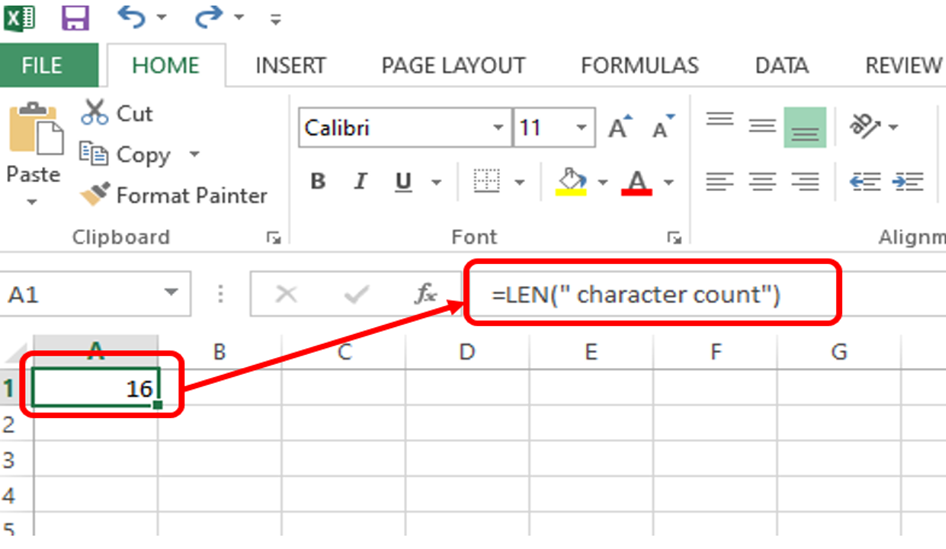To count the number of characters in a text present in another cell you can give the cell number in the argument of this formula and it will show you the number of characters (including spaces) present in the cell.

=LEN(A1)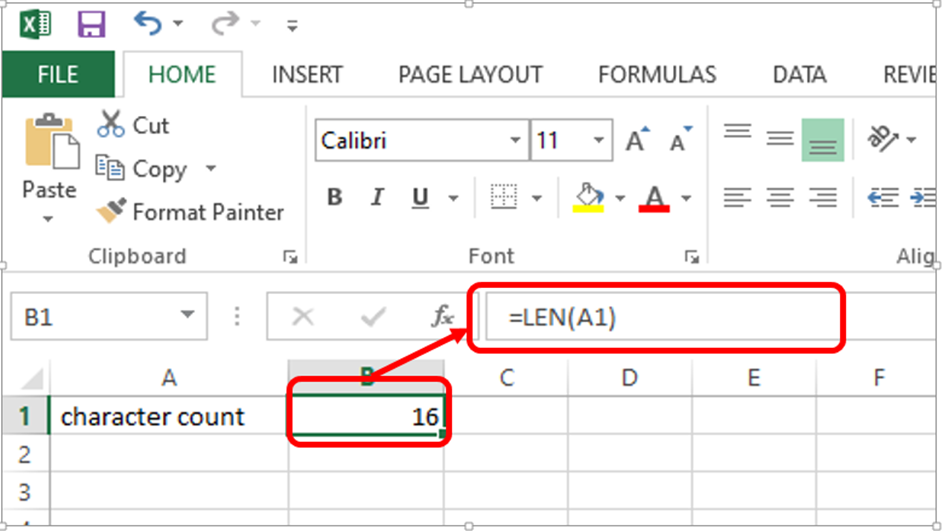### Find the Character Count of a Range of Cells in Excel

So far so good right?

Now what to do if you need to find the character count of a range of cells? A whole column, row, or maybe a whole sheet?

Well, we use a formula for that as well.

We will use LEN in combination with SUMPRODUCT. SUMPRODUCT is a function that is used to add arrays instead of numbers. So LEN of each cell will be an array which SUMPRODUCT will add for us.

=SUMPRODUCT(LEN(range))

To count the number of characters in a text present in a range of cells, we will give cell range in the argument of this formula.

=SUMPRODUCT(LEN(A1:A3))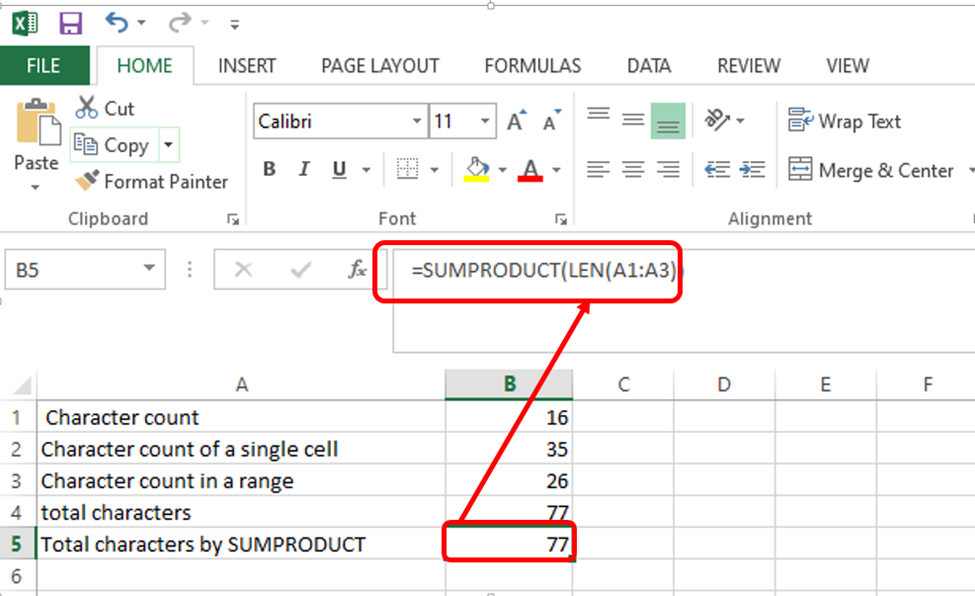This gives us the total number of characters which matches the sum of the individual cell's character count.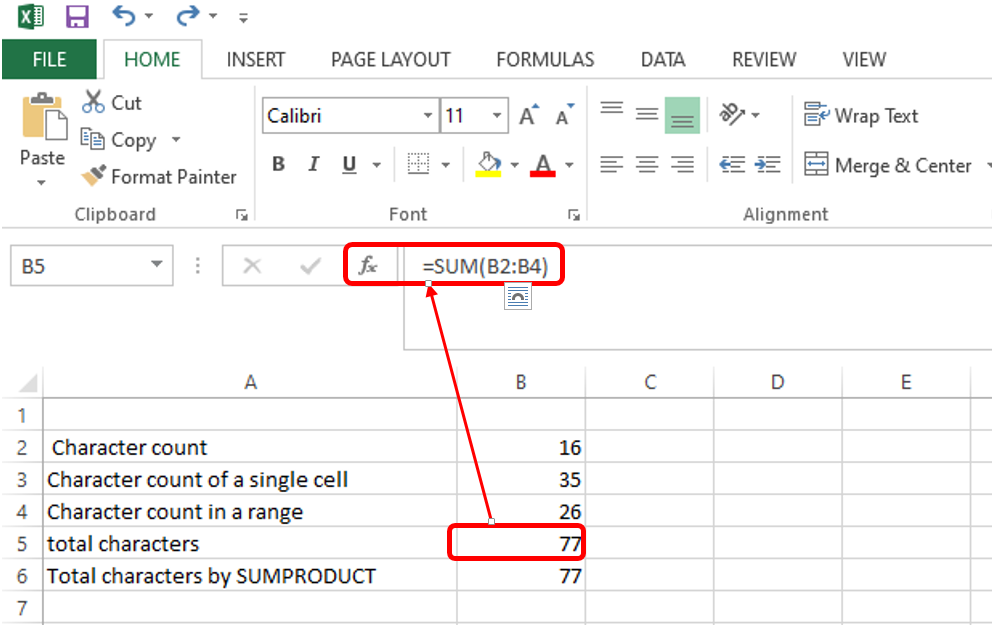### Find the Character Count without spaces in MS Excel:

Sometimes we need to know the character count of our text without the spaces. To get this number we will use the SUBSTITUTE function along with the previously used LEN function.

What does the substitute function do?

On its own a substitute function, as its name suggests, replaces a character or string of characters with another in the range selected. The substitute function takes the following four arguments:

=SUBSTITUTE (text to search, search for, replace with, [occurrence number])

Text to search is the cell you want the function to work on. "search for" is the character you want to replace, "replace with" is the character or string you want the previous argument to be replaced with. "Occurrence number" is an optional argument, used to indicate the number of instances to be replaced.

Now how can we use this function in our formula to get character count without spaces?

=LEN(SUBSTITUTE(Cell," ",""))

This formula will first omit the blank spaces using the substitute function and then count the characters with the LEN function.

=LEN(SUBSTITUTE(A2," ",""))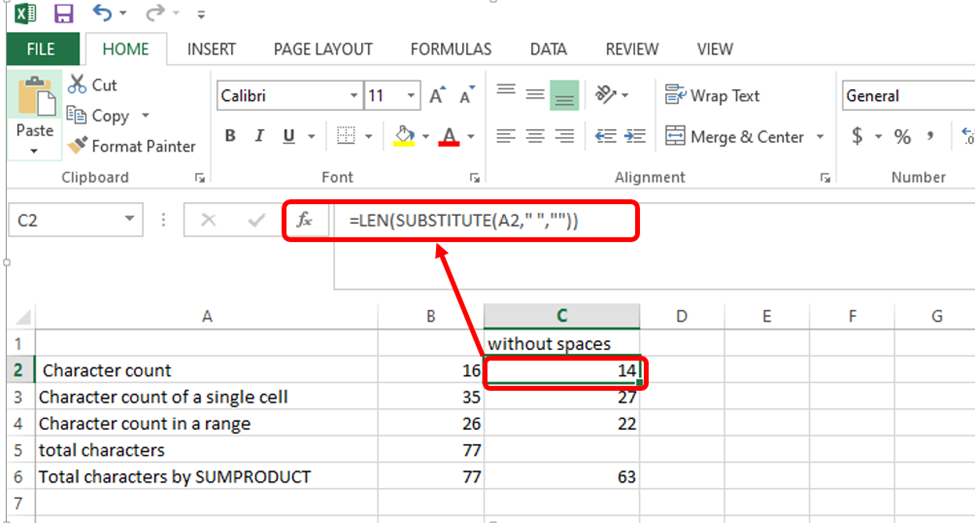Now to find the number of characters in a range of cells, we will combine the SUMPRODUCT, SUBSTITUTE, and LEN formulas together:

=SUMPRODUCT(LEN(SUBSTITUTE(A2:A4," ","")))

This formula will remove the blank spaces from each cell in the range, count the number of characters, and then treat each cell in the given range as an array. It will then sum these arrays giving us the total number of characters in the given range.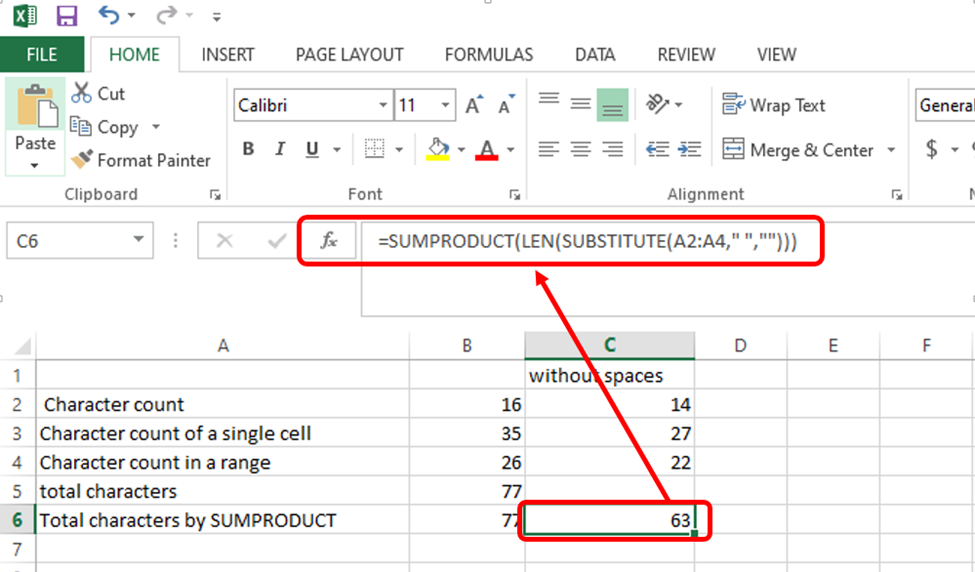And there you go, now you know how to find the number of characters in excel. Hope you found this article interesting and informational!

### Conclusion

Microsoft Excel is a great application that has a lot of options and formulas to give you a character count. If that doesn't work, or you want another way to count characters feel free to copy and paste your text into the character counter on our home page.